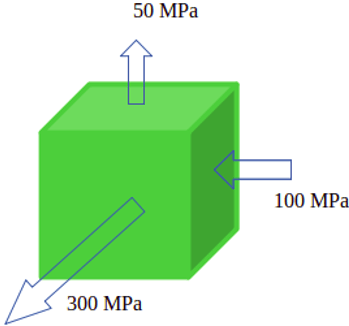Q.1
##### Physical Meaning of von-mises yielding criteria is that yielding occurs when the _____________ reaches a critical value.
• a) distortion energy
• b) strain energy
• c) potential energy
• d) kinetic energy
Q.2
• a) True
• b) False
Q.3
##### Von-mises or Distortion-energy Criteria proposed that yielding will occur when the second invariant of the stress deviator J2 exceeds some critical value. The second invariant of the stress deviator J2 is equal to __________
• a) 1/6 [(σ1 – σ2)2+(σ2 – σ3)2+(σ3 – σ1)2]
• b) 1/2 [(σ1 – σ2)2+(σ2 – σ3)2+(σ3 – σ1)2]
• c) [σ1 + σ2 + σ3]
• d) 1/2 [(σ1 – σ2)+(σ2 – σ3)+(σ3 – σ1)]
Q.4
• a) σo
• b) σo/2
• c) σo/3
• d) σo/√3
Q.5
• a) True
• b) False
Q.6
##### Stress analysis of structural material for the submarine gives the state of stress as shown in the figure. The yield strength of the material isMPa. Using Von-mises yielding criteria determine whether yielding will occur or not? If not, what is the factor of safety?• a) Yielding will not occur
• b) Yielding will occur, and the factor of safety is 2.5
• c) Yielding will occur, and the factor of safety is 1.53
• d) Yielding will occur, and the factor of safety is 1.28
Q.7
• a) τ
• b) τ/3
• c) τ/√3
• d) τ/2
Q.8
• a) τ = σo
• b) τ = σo/2
• c) τ = σo/3
• d) τ = σo/4
Q.9
• a) k = σo
• b) k = σo/2
• c) k = σo/3
• d) k = σo/4
Q.10
##### Stress analysis of structural material for the submarine gives the state of stress as shown in the figure. The yield strength of the material isMPa. Using Tresca’s yielding criteria determine whether yielding will occur or not? If not, what is the factor of safety?• a) Yielding will not occur
• b) Yielding will occur, and the factor of safety is 1.125
• c) Yielding will occur, and the factor of safety is 1.53
• d) Yielding will occur, and the factor of safety is 1.28